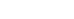# Charts of RealVol Daily Indices

• Symbol Guide.
• Select the desired chart below. Instructions can be found below the charts.
• Click on the colored pennants in the aqua chart headers to turn individual graphs on or off.
• Mobile devices are limited to two years of data for performance reasons.
• Hover the mouse over points to see their values in the body of the chart and in the aqua headers.
• To delete or restore a line, click on its corresponding-colored pennant in the aqua headers.

VOLS: RealVol SPY Indices

Chart Type:

Sources of Underlying Data: NYSE Arca

### Select Volatility Chart Definitions and Instructions

• URP:  The underlying reference price.  For example, if the underlying is SPY, then SPY closing prices are the URPs.
• HVOL, RVOL, and lagged VOLs and DVOLs ("Forecast & Actual"):

Each method of forecasting (RVOL and HVOL) comprises six time periods, corresponding to forecasts of one-day (“d”), one-week (“w”), one-month (“m”), one-quarter (“q”), one-half-year (“h”), and one-year (“y”), realized volatility, the latter being measured by DVOL for 1- and 5-day periods and VOL for the four remaining longer time intervals (see note below).  For any given forecast on any day, the resulting actual volatility for the ensuing time period is lagged by the appropriate number of trading days (ranging from 1 to 252) so that it can be compared graphically to the forecast to which it corresponds.  So, for example, on any given day, the RVOLm (forecast) would be plotted alongside the VOLm index 21 trading days later (actual).

The second chart, Percentage Error, depicts the residual of the actual volatility when compared to the forecast.  A value above the horizontal 0.00 axis indicates a forecast that overestimated the resulting volatility, while values below zero show periods when the forecast underestimated the actual volatility.  The values are expressed as a percentage error, calculated by subtracting 1 (100%) from the ratio of the forecast divided by the realized volatility.  For example, if the forecast were 25% and actual volatility turned out to be 20%, the percentage error would be (25%/20%) - 1 = 1.25 - 1 = 0.25 (25%). Note again, that because of the need for more data points to properly calculate VOL than are needed to estimate DVOL, the latter is used for comparison to the 1- and 5-day HVOLs and RVOLs.

The orange histogram plots, for the historical time period studied, the number of observances of each magnitude of error, placed in various "bins" along the horizontal axis.  The more errors that fall into the central bins, and the "tighter" the bell curve, the more accurate the forecast is considered to be. (Note: Regardless of the time period selected for the forecast, the histograms incorporate all available historical data for the underlying asset.)